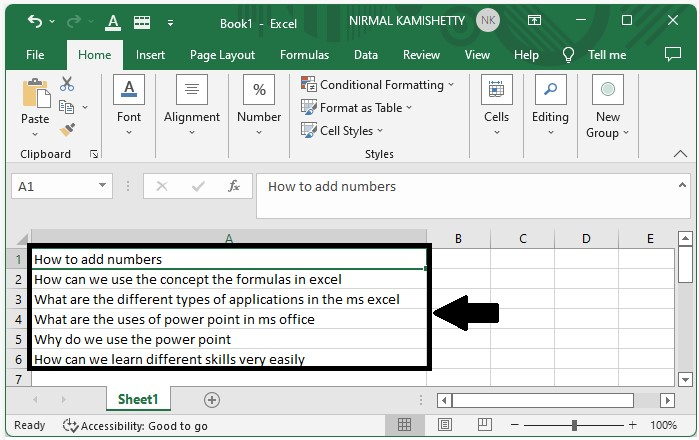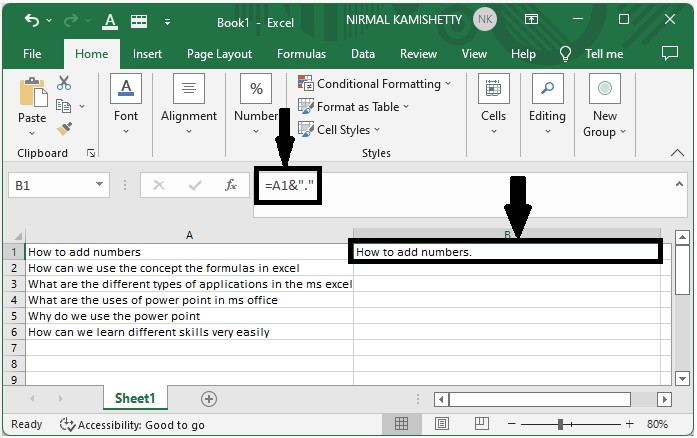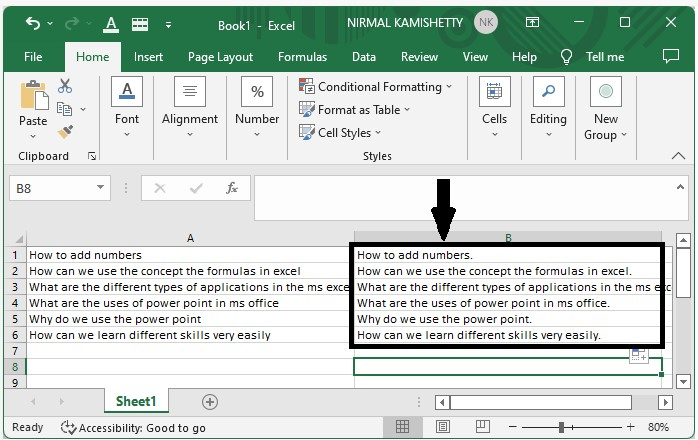# How to Add Periods to The End of Cell Contents in Excel?

Have you ever thought how you can use the features available in Excel to automatically add a period mark at the end of multiple cells? A Period is nothing but a dot(.) symbol which we use to represent the end of a sentence. Mostly when we write or type any sentence in Excel or Word, we tend to forget the full-stop at the end of the text or even after the end of sentences. In this tutorial, we will show how you can add period marks at the end of cell contents in Excel.

## How to Add Periods at the End of Cell Contents in Excel

Let us take a simple example to show how you can add Periods at the end of cell contents. The period which we will be adding at the end of cell contents is a dot(.). Here we will use a formula to add Periods at the end of cell contents in Excel.

### Step 1

Let us assume we have an Excel sheet that contains a list of sentences, as shown below −To add period marks at the end of cell contents, click an empty cell. In our case, we selected the cell "B2" and then enter the formula as ADDRESS OF DATA&"." In our case, it will be A1&".". Then press the Enter button to get the first result, as shown below### Step 2

To get all the other results, drag down from the first result till all the results are filled successfully. The final output will look like the one shown below −## Conclusion

In this tutorial, we used a simple example to demonstrate how you can use the features available in Excel to add period marks at the end of cell contents in an Excel sheet.